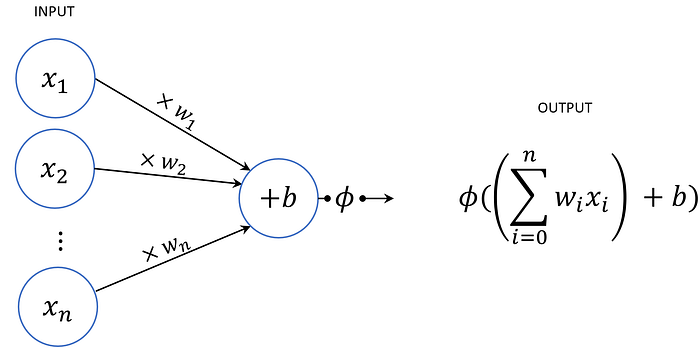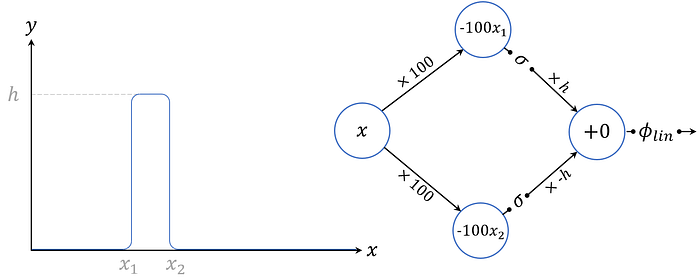# Understanding Neural Networks

Jul 2, 2021 11:17:24 AM

Neural networks (NNs) have always appealed to me. Not solely because they are an abstract representation of the human brain, but also due to their achievements over the years. Handwriting recognition, self-driving cars, and outperforming humans in Go, what will be next on the list?Calculation of a neuron where φ represents an arbitrary activation function.

Studying the basics of NNs, one comes across the fairly clean notion of a neuron. Chaining these neurons forms a NN, so it’s nothing more than some weighted sums combined with activation functions. It puzzles me how on earth a construction like this is able to recognise faces. In order to get a better understanding, I tried to create a NN that would calculate f(x) = x². I tried several “architectures” but they never gave the desired result…

Neural networks can do it all
My unsuccessful attempt in approximating x² made me question what functions can actually be calculated with a NN. It only consists of basic operations, so there must be some limitations, right? Well, hold your chair, because you’re about to get blown away. It did not take much research until I came across “the Universal Approximation Theorem”, which posits that any function can be approximated by a NN!

Despite the astonishment of the result, the proof is surprisingly easy to grasp. Which can mainly be attributed to Michael Nielsen, who goes over the proof in a very understandable fashion. In his construction he starts with a NN that outputs a bar with a variable domain, height and precision.The bar function on the left is the output of the NN on the right. Here, σ is the usual sigmoid activation and φₗᵢₙ(x) = x is the lineair activation.

Every function can be approximated with multiple bars by iterating this construction. The result looks very similar to the more widely known approximation of the integral.

What is the catch?
Even though not much details have been given, this example already exposes some defects. First of all, there is a continuity assumption on the given function, so it can’t make sudden jumps. In most real life use cases, however, a continuous approximation suffices, so this is not really a limitation.

Secondly, the theorem promises an approximation; each bar mimics the function closely but not exactly. This means that the NN will still have an error margin greater than zero. Luckily this error can be minimized by just increasing the number of bars (and therewith the size of the network).

Lastly, the construction only covers a limited domain. Hence, it can’t replace X² on all the real numbers, but only in-between a and b. I like to draw the analogue with the fact that NNs can’t extrapolate outside their trainset. It is a supervised machine learning algorithm after all.

Now a little more practical
It’s always good to pay attention to the little caveats, but overall I was very impressed by the implications of this theorem. Mathematicians are pleased knowing a solution exists without exactly finding it. It’s been quite some time, however, since I was studying maths and in my day-to-day job I’m asked to deliver practical results rather than theoretical ideas. So, I continued my research.

The discussed construction has no restrictions on the size of the NN and its weights. Moreover, it’s completely different from our usual approach of converging to a NN solution. The field of computability theory, however, tries to capture the compute power of NNs as a function of their size. I discovered that this field has a lot more open questions, but, luckily, also some pretty results.

One of these is the fact that the unbounded weights in NNs can be replaced with polynomial ones by increasing the depth by just one layer, where the size of this last layer is also polynomial. Here, polynomial means polynomial in the size of the input vector (Goldmann, Håstad, & Razborov, 1992). This tells us that we don’t need gigantic NNs, and it even turns out that many functions are computable by NNs of polynomial size and constant depth.

This gave me enough confidence to try to approximate X² once more. Here you can find my last result. I hope you enjoyed a little background information about NNs and please let me know if you have found a better approximation!